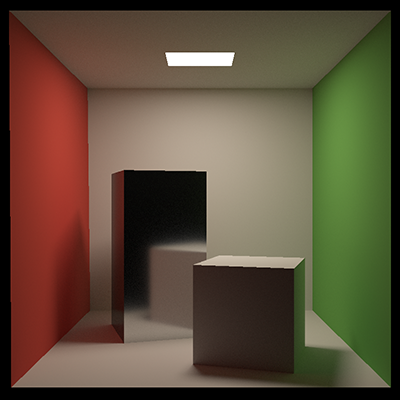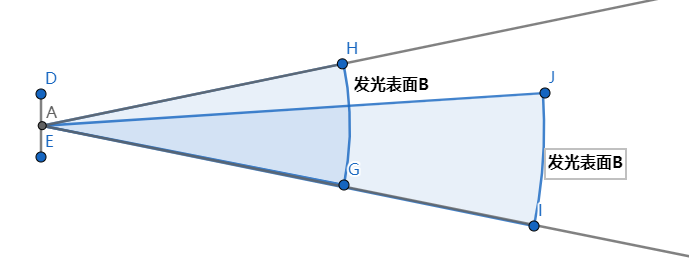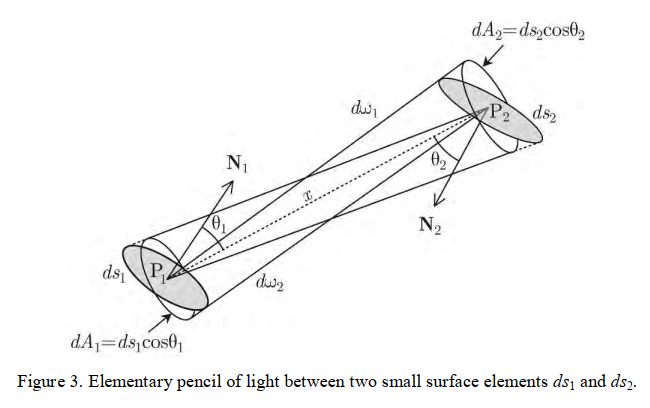一些对辐射度量学的理解回忆在写path tracing时，代码并没有刻意去计算距离对光线能量的衰减。

那么衰减到底在哪里体现的呢？

先看一下定义：d\omega_2dA_2\cos\theta_2

= \frac{ds_1\cos\theta_1}{r^2}dA_2\cos\theta_2

= ds_1\cos\theta_1\frac{dA_2\cos\theta_2}{r^2}

= ds_1\cos\theta_1d\omega_1